Courses
Courses for Kids
Free study material
Offline Centres
More

# Chemical Bonding and Molecular Structure Chapter - Chemistry JEE MainLast updated date: 01st Dec 2023
Total views: 21.3k
Views today: 0.21k## Concepts of Chemical Bonding and Molecular Structure for JEE Main Chemistry

We'll look at the structure of atoms as well as the subatomic particles that make up atoms in this chapter. To understand the bond formation, one must first appreciate the electronic structure of atoms or the arrangement of electrons around the central nucleus.

This chapter is one of the most important, simple, and high-scoring for competitive exams like JEE Main 2023, and mastering it will help you do well on the exams.

## JEE Main Chemistry Chapters 2024

### Important Topics of Chemical Bonding and Molecular Structure Chapter

• Chemical Bonding

• Fajan’s rule

• Lewis Dot Structures

• Formal charge

• Hybridization

• Valence Bond Theory

• Molecular Orbital Theory

• VSEPR Theory

### Chemical Bonding

The force of attraction between the atoms or ions of molecules occur due to sharing of electrons or by transfer of electrons from the atoms to complete octet and attain stability.

### Valence Shell Electrons

The outer shell electrons that take part in chemical bonding are known as valence shell electrons.

### What is Chemical Bonding and Molecular Structure?

The study of chemical links between atoms or molecules is known as chemical bonding. This chapter discusses why only particular atoms can combine to form a new product and why they must be arranged in a specific shape. VSEPR, valence bond theory and molecular orbital theory are some of the theories that can explain all of the occurrences in detail. Bonding isn't simply an example; it's nature's way of bringing every atom or molecule to the most stable state possible.

Every structure in the universe is the consequence of the development of specific types of bonds. In reality, bonding is nothing more than the joining of two atoms.

### Classification of Chemical Bonds

During the formation of chemical bonding, the materials participate in producing compounds. The firmness of the subsequent compound can be evaluated by the type of chemical bonds it comprises.

The different types of chemical bonds formed vary in strength and properties.

There are 4 main classifications of chemical bonds that are shaped by atoms or molecules to yield compounds.

• Ionic Bonds

• Covalent Bonds

• Hydrogen Bonds

• Polar Bonds

1. ### Ionic Bonding

This type of chemical bonding contains a relocation of electrons from one atom or molecule to the alternative atom. At this time, an atom misses an electron, which is in sequence added by a neighboring atom.

As soon as such electron transfer happens, one of the atoms creates a negative charge and is named the anion. The additional atom cultivates a positive charge and is named the cation.

1. ### Covalent Bonding

The covalent bond specifies the distribution of electrons between atoms. The compounds that consist of carbon (also known as organic compounds) usually display this sort of chemical bonding. The duo electrons, which are united by the two atoms, now spread around the nuclei of atoms, prominent to the formation of a molecule.

1. ### Polar Covalent Bonding

The nature of the covalent bonds is that they can either be Polar or Nonpolar. The electrons are united unevenly in polar covalent chemical bonding as the additional electronegative atom attracts the electron nearer to itself and away from the fewer electronegative atoms.

1. ### Hydrogen Bonding

If you make some comparison between ionic and covalent bonding, hydrogen bonding turns out to be a fragile procedure of chemical bonding. This kind of polar covalent bonding between oxygen and hydrogen is that the hydrogen develops a partial positive charge in the formation of the water.

### Kossel – Lewis Approach to Chemical Bonding

A Chemical bond is an attraction force between atoms of a molecule.

In 1916 Kossel and Lewis succeeded in explaining the chemical bonding in terms of electrons.

### Octet Rule:

The octet rule states that the atoms combine either by transfer of valence electron(s) or by sharing of valence electron(s) to form molecules by completing the octet of electrons in their valence shell to attain the nearest noble gas configuration (hydrogen element completes its duplet to attain the configuration of helium).

• Limitations of Octet Rule:

1. Incomplete Octet: The central atom of some molecules have incomplete octet i.e less than eight electrons in their valence shell. This is generally observed in the molecule of elements which has less than four valence electrons.

Example: BeCl2 (2 valence shell electrons in Be), BF3(3 valence shell electrons in B), LiH  (1 valence shell electron in Li).

1. Odd-Electron Molecules: The molecules which are formed from the atom containing odd electrons in the valence shell.

Example: Molecules of nitrogen- Nitric oxide (NO) and nitrogen dioxide (NO2).

1. Extended Electron: The molecules of some elements contain more than eight electrons in the valence shell of central atoms. The elements of the 3rd period and beyond have d orbitals which also participate in the chemical bonding.

Example: BrCl3 XeF2, PCl5, SF6

1. Noble Gas Compounds: Noble gases have complete octet but some noble gases such as xenon and krypton form compound with fluorine and oxygen.

Example: XeOF2, XeF6, KrF2, XeO3

### Lewis Dot Structures:

G.N. Lewis proposed the notations to represent the elements with their valence electrons. The valence electrons are represented by the dot around the symbol of the element.

Example: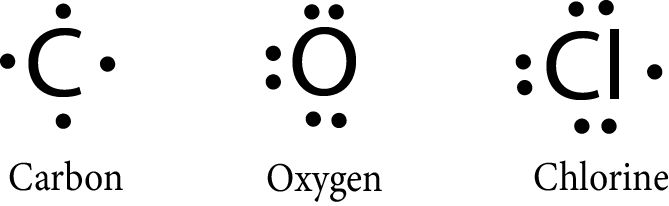The molecules can also be represented by the lewis symbol notations in which:

• Each atom shares a single electron to form an electron pair.

• Each electron pair represents the bond.

• The atoms combine to attain the noble gas configuration and each atom should  have 8 electrons around it.

Example: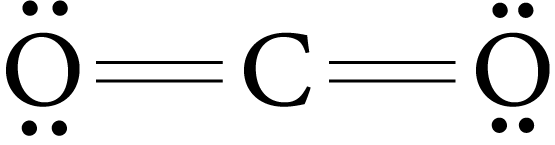Lewis dot structure of Cl2 molecules.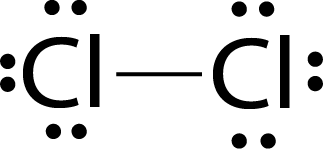• The single electron pair between two atoms represents a single covalent bond.

Example: H2O, NH3

• The two-electron pairs shared between two atoms represent the double bond.

Example : O2, CO2

• The three electron pairs shared between two atoms represent the triple bond.

Example: CO, C2H2, N2

### Covalent Bond:

The covalent bond is formed by sharing of electron (s) between two atoms. Atoms combine to become stable by attaining the nearest noble gas configuration.

A single covalent bond is formed when a single electron pair is shared between the atoms, a double covalent bond is formed when two electron pairs are shared, and a triple bond is formed when three electron pairs are shared.

### Formal Charge:

The formal charge is the charge on each atom of the molecule or ion. The ions are represented by the net charge possessed by the ion whereas the formal charge is the charge possessed by each atom in the molecule or ion. In lewis's structure, the atoms in molecules or ions are represented by their formal charge.

The formal charge of an atom in a molecule or ion is given by the formula:

Formal Charge  =   V - N - ½  (B)

where

V: Total number of valence electrons present in the free atom

N: Total number of nonbonded electrons or lone pairs

B: Total number of bonded or shared electrons

The molecules in which the atoms possessing the smallest formal charge are considered to have the lowest energy and more stability.

### Ionic or Electrovalent Bond:

Ionic or electrovalent bond is formed by the transfer of electron(s) from one atom to another to become stable by attaining the nearest noble gas configuration.  The loss of electron(s) from the atom forms a positively charged ion is known as cation and the gaining of electron(s) forms a negatively charged ion known as an anion. There is a strong electrostatic force of attraction between cations and anions, this force is the ionic bond.

Conditions for the formation of ionic bonds:

• Low ionization enthalpy of cation

• High electron gain affinity of anion

• High lattice energy of ionic crystal

Ionisation Enthalpy: It is the amount of energy required to remove loosely bound electrons from an isolated gaseous state of an atom.

M(g)   $⟶$$\longrightarrow$    M+(g) +  e

Electron Gain Enthalpy: It is the amount of energy released when an electron is gained by the gas phase atom in its ground state.

X(g)   +  eー      $⟶$$\longrightarrow$    X(g)

Lattice Enthalpy: The amount of energy required to completely separate one-mole ionic crystal into its constituent ions in the gaseous state is known as lattice enthalpy of an ionic crystal. The more the lattice enthalpy of ionic crystal, the more is the stability of ion ionic crystal.

The factor on which the lattice enthalpy of the ionic crystal depends:

• Size of ions: Smaller the size of ions more will be the lattice enthalpy.

• Charge on ions: Greater the charge on the ions stronger will be interionic attraction and hence more will be the lattice enthalpy.

### Bond Parameters:

• Bond Length:

1. It is the equilibrium distance between the nuclei of the two bonded atoms in a molecule.

2. The bond length of a covalent molecule is determined by the covalent radii of each bonded atom.

3. The bond length order of single, double and triple bond:

Triple bond  <  double bond  <  single bond

• Bond Angle:

1. It is the angle between the orbitals of molecules containing the bonded electron around the central atom.

2. It helps in determining the shape of the molecule.

3. It is expressed in degrees.

The factor that affects the bond angle:

•  Bond angle is proportional to the electronegativity of the central atom and inversely proportional to the electronegativity of the peripheral atoms.

• Bond angle is proportional to the percentage of s character of the hybridised orbitals and inversely proportional to the percentage of p character of the hybridised orbitals.

• The bond angle is proportional to bp-bp repulsion and inversely proportional to lp-bp and lp-lp repulsion.

• The bond angle is proportional to the size of peripheral atoms of the molecule.

• Bond Enthalpy:

1. It is the amount of energy required to break one mole of a particular type of bond between the two atoms of a molecule in a gaseous state.

2. The more the bond enthalpy, the more will be the more stability of the bond.

3. For polyatomic molecules, the bond enthalpy is the average or mean bond enthalpy of all the bonds in the molecule.

The factors affecting the bond enthalpy:

• The chemical environment of the bond is to be broken.

• The more the number of bonds to break the more will be the bond enthalpy. The order of bond enthalpy:

Triple bond  >  Double bond  >  Single bond

• Bond Order:

1. Bond order is the number of electron pairs or bonds present shared between two atoms of a molecule.

2. For single bond: one electron pair is shared between two atoms → bond order = 1

For double bond: two electron pairs are shared between two atoms → bond order = 2

For triple bond: three electron pairs are shared between two atoms → bond order = 3

1. Isoelectronic structures of molecules or ions have the same bond order.

• Resonance Structure:

1. The resonance structure is the group of Lewis structures formed due to the delocalization of electrons in a polyatomic molecule or ion.

Example: benzene and CO32-

1. The single lewis structure of the molecule could not explain the properties of some polyatomic molecules or ions, which is the implication that the molecule has resonance structures.

• The Polarity of Bonds:

1. The bond formed between two different atoms (or heteronuclear atoms) of the molecule has polarity in a bond due to the difference in electronegativity of different atoms and is hence known as a polar bond. Example: HCl, H2O

2. The polarity in bonds gives rise to the dipole moment in the molecule. The dipole moment in the molecule.

• Dipole Moment:

1. The dipole moment is the product of charge (Q) and distance of separation between the atoms(r).

2. The dipole moment is a vector quantity and it is denoted by the symbol μ.

3. The unit of dipole moment is debye (D).

4. The resultant dipole moment of a molecule helps in determining the shape and polarity of a molecule.

Significance of Dipole Moment - The molecules having zero moment are non-polar molecules and those having μnet ≠ 0  are polar in nature.

The value of the dipole moment can be used for determining the amount of ionic character in a bond. The formula for determining percentage of ionic character is given below –

Percentage of ionic character = $\dfrac{\text{Experimental value of dipole moment}}{\text{Theoretical value of dipole moment}} \times 100$

### Fagan's Rule:

It states that the covalent bond has some partial ionic character whereas the ionic bonds have partial covalent character. The partial covalent character in an ionic bond can be measured in terms of the following Fajan’s rule:

• The smaller the size of the cation and greater the size of the anion the more will be the covalent character in the ionic bond.

• The cations with greater charge contribute to the more covalent character in the ionic bond.

• Transition metal cations are more polarising than the cation with a noble gas configuration with the same charge and size as that of transition metal cations.

For the ionic character in covalent bond, the conditions will be opposite on the covalent characters in the ionic bond.

### Valence Shell Electron Pair Repulsion (VSEPR) Theory:

This theory helps in determining the shape of the molecule of covalent compounds on the basis of the repulsion of the lone pair and bond pairs in the free spatial arrangement around the central atom of the molecule. The postulates of the VSEPR are as follows:

• Only valence shell electron pairs around the central atom contribute to the shape of the molecule.

• The electron pair repels each other as these are negatively charged due to their negatively charged electron cloud.

• Electron pairs occupy the spherical space around the central atom such that they are at maximum distance from each other and have minimum repulsion between them.

• Multiple bonds are treated as a single electron pair.

The repulsion order of bond pair(bp) and lone pair(lp) is:

lp-lp  >  lp-bp > bp-bp

### Hybridisation:

• It is the mixing of two orbits of nearly different energy levels to get the same number of orbitals of the equivalent energy and shape.

For example: The 1s and 2p orbitals of the same shell hybridize to give 3 orbitals of sp2 hybridisation.

• Number of hybrid orbitals can be determined by the formula:

Number of hybrid orbitals (X)  =   ½ (Total valence electron of central atoms) - Total number of monovalent atoms - charge on cation + charge on the anion.

Or

Number of hybrid orbitals (X)   =  number of bond pairs  +  number of lone pairs on central atoms

• Hybridisation helps in the determination of the geometry of molecules. The bond pairs and lone pairs both are responsible for the geometry of the molecule whereas only bond pairs are responsible for the shape of the molecule.

### Types of Hybridization –

Hybridization takes place by involvement of various orbitals such as s, p, and d orbitals. It has following types -

• sp Hybridization – one s and one p orbitals take part in hybridization and each sp hybrid orbital has 50% s - character and 50% p – character. Two sp- hybrid orbitals are formed.

• sp2 Hybridization – one s and two p orbitals participate in this hybridization and form three hybrid orbitals. Each of these hybrid orbitals show 33% s character and 67% p character.

• sp3 Hybridization – one s and three p orbitals participate in this hybridization and form four hybrid orbitals. Each of these hybrid orbitals show 25% s character and 75% p character.

• dsp2 Hybridization – one - s, two p and one d orbitals participate in this hybridization and form four hybrid orbitals.

• sp3d Hybridization – one s, three p and one d orbitals participate in this hybridization and form five hybrid orbitals.

• sp3d2 Hybridization – one - s, three p and two d orbitals participate in this hybridization and form three hybrid orbitals.

• d2sp3 Hybridization – one - s, three p and two d orbitals participate in this hybridization and form three hybrid orbitals.

 S. No. Atomic Orbitals Used Hybrid Orbitals Formed Bond Angle Geometry Example 1. s, p Two sp orbitals 180° Linear C2H2 2. s, p, p Three sp² orbitals 120° Planar trigonal BCl3 3. s, p, p, p Four sp³ orbitals 109.5° Tetrahedral CH4 4. d, s, p, p Four dsp² orbitals 90° Square planar [Ni(CN)4]²¯ 5. s, p, p, p, d Five sp³d orbitals 90° and 120° Trigonal bipyramidal PF5 6. s, p, p, p, d, d Six sp³d² orbitals 90° and 120° Square pyramidal BrF5 7. d, s, p, p, p Five dsp³ orbitals 90° T — shaped ClF3

### Valence Bond Theory:

This theory states that, when the two atoms are brought closer to each other, there are some attractive forces as well as some repulsive forces acting between the two atoms. When the bond is formed, the net attractive force is balanced by the repulsive forces.

Let us consider two hydrogen atoms which contain nuclei NA and NB and electrons in the valence shell as eA and eB. When the two atoms are brought closer, the attractive forces- NA - eA  and  NB - eB  ; also NA - eB and   NA - eA  acts between the atoms whereas the repulsive forces- NA -NB and eA and eB also act at the same time. A stage is reached when the net attractive force is balanced by net repulsive force, and the bond between two hydrogen atoms is formed.

### Types of Covalent Bond:

There are two types of covalent bonds:

• Sigma (σ) Bond: This type of covalent bond is formed by the head-on overlapping of two atomic orbitals along the internuclear axis.

1. s-s Overlapping: The two half-filled s-orbitals of the two atoms overlap along the internuclear axis.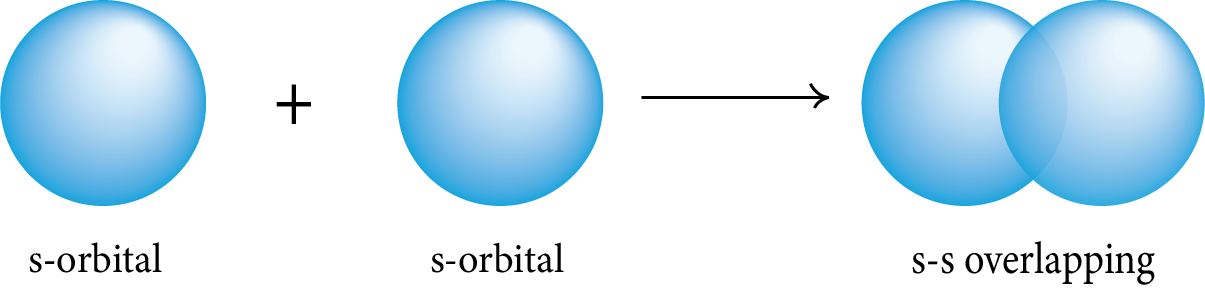1. s-p Overlapping: The half-filled s-orbital of one atom overlaps with the half-filled p-orbital of another atom along the internuclear axis.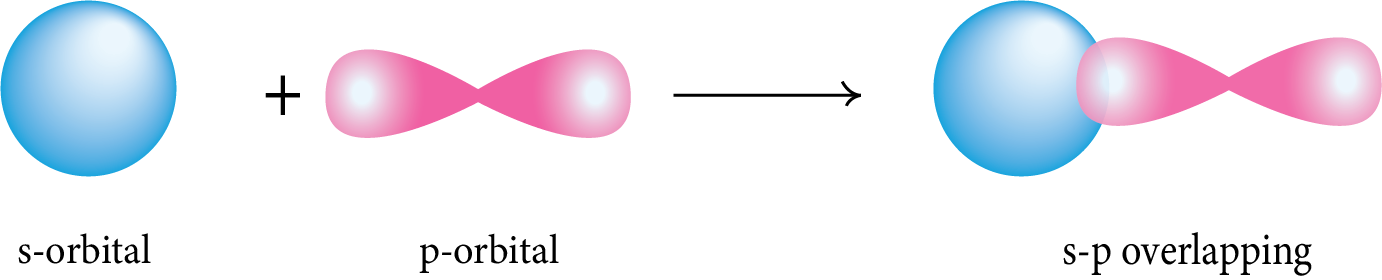1. p-p Overlapping: The half-filled p-orbital of one atom overlaps with the half-filled p-orbital of another atom along the internuclear axis.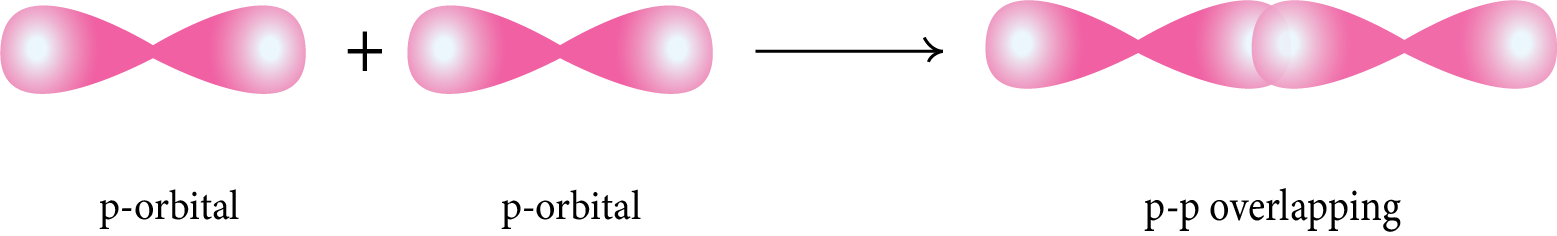• Pi (π) Bond:  This type of covalent bond is formed by the lateral or sidewise overlapping of two atomic orbitals perpendicular to the internuclear axis.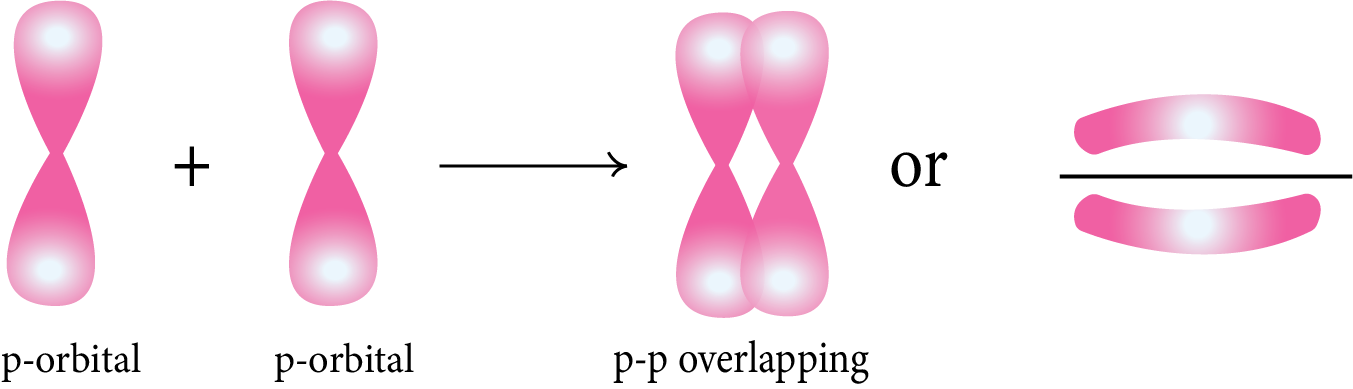### Strength of Sigma (σ) and pi(π) Bond:

• Sigma (σ) bond is formed by the head-on overlapping whereas the pi (π) bond is formed by sideways overlapping of the orbital.

• The extent of overlapping is greater in the sigma (σ) bond than in pi (π) bond. Therefore, the strength of the sigma (σ)bond is more than the pi (π) bond.

• In multiple bonds between the atoms, pi bonds are formed in addition to the presence of sigma bonds.

### Molecular Orbital Theory (MOT):

• According to MOT, the atomic orbital of the atoms combines with a linear combination of atomic orbital (LCAO) to form the molecular orbitals.

• The addition of atomic orbital gives rise to the bonding molecular orbital whereas the subtraction of atomic orbitals gives rise to the antibonding molecular orbital. The antibonding MOs are represented by (*) and they have more energy than bonding MOs.

ψMO =  ψA   ±  ψB

Bonding MOs:            σ 2s,  σ2pz, π2py, π2px

Antibonding MOs:    σ* 2s,  σ*2pz,  π*2py  π*2px

Electronic Configuration of Molecule:

The distribution of electrons in the orbital of molecules in the increasing order of energy is known as the electronic configuration of molecules.

• The electronic configuration of molecules which have more than 14 electrons such as O2 has 16 electron, have electronic configuration as follows:

σ 1s2,  σ* 1s2, σ 2s2,  σ* 2s2, σ2pz2 (π2py2=  π2px2) , (π*2py1 =π*2px1)

• The electronic configuration of molecules which have equal to or less than 14 electrons such as N2 has 14 electrons, have electronic configuration as follows:

σ 1s2,  σ* 1s2, σ 2s2,  σ* 2s2, (π2px2=  π2py2), σ2pz2

Bond Order:

Bond order is half of the difference of the electrons in bonding orbital and antibonding orbitals.

B.O.  =  ½ (Nb - Na)

where, Nb = Total number of electrons in bonding orbital

Na = Total number of electrons in antibonding orbital

Example : O2

B.O. (O2)= (10 - 6)/2  =  4/2  =  2

The O2 molecule has 2 bonds between each O atom.

Magnetic Properties:

The molecule is said to be diamagnetic if all the electrons in the molecular orbitals are paired. The molecule is said to be paramagnetic if the electron has at least one unpaired electron in the molecular orbital.

Example: O2 molecule is a paramagnetic in nature as it has 2 unpaired electrons in each in the  π*2py1 and  π*2px1

Spin only magnetic moment can be calculated by the formula:

μs = $\sqrt{n\left(n+2\right)}$$\sqrt{n(n + 2)}$

where,  n : Number of unpaired electrons

### Hydrogen Bonding:

When the highly electronegative elements such as fluorine, nitrogen and oxygen, are bonded covalently with the H atom, the electron of a hydrogen atom is slightly shifted towards the highly electronegative atom, which develops a partial positive charge on H atom. The partial positive charged hydrogen atom is attracted towards the electronegative atom causing hydrogen bonding.

There are two types of hydrogen bonding:

• Intermolecular Hydrogen Bonding: The hydrogen bonding is formed between two molecules of the same or different compounds.

Example: H2O, HF

Hδ+-Fδ-……Hδ+-Fδ-…Hδ+-Fδ-…Hδ+-Fδ-

• Intramolecular Hydrogen Bonding: The hydrogen bonding is formed when the highly electronegative (O, F, N) atom in the molecule is attracted to the hydrogen atom bonded to the electronegative atom (O, N, F) within the molecule.

Example: o-nitrophenol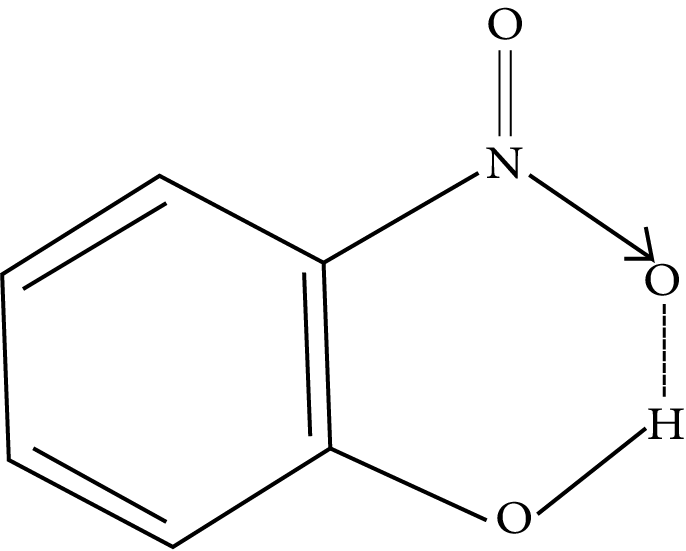### JEE Main Chemical Bonding and Molecular Structure Solved Examples

Example 1:  Hybridisation state of chlorine in ClF3 is :

1. sp3

2. sp3d

3. sp3d2

4. sp3d3

Solution: (b)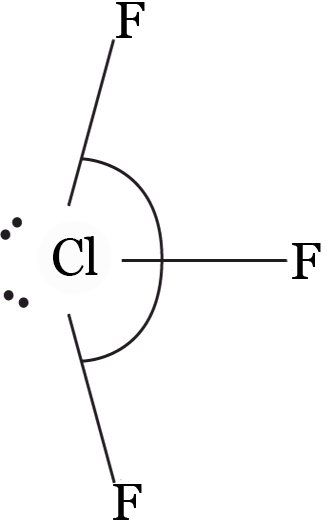Bond pairs = 3, lone pairs = 2    ⇒   hybridisations = sp3d

Key Points:

Number of hybrid orbitals   =  number of bond pairs  +  number of lone pairs on central atoms

Example 2: As the s-character of hybridised orbital increases, the bond angle

1. increase

2. becomes zero

3. does not change

4. decrease

Solution:  (a) increase

The bond angle increases with increase in percentage of s character of the hybridized orbitals.

Key Points:

The factor that affects the bond angle:

• Bond angle is proportional to the electronegativity of the central atom and inversely proportional to the electronegativity of the peripheral atoms.

• Bond angle is proportional to the percentage of s character of the hybridized orbitals and inversely proportional to the percentage of p character of the hybridized orbitals.

• Bond angle is proportional to bp-bp repulsion and inversely proportional to lp-bp and lp-lp repulsion.

• Bond angle is proportional to size of peripheral atoms of molecule.

Example 3: Using MO theory, predict which of the following species has the shortest bond length?

1. O2

2. O22—

3. O22+

4. O2

Solution: (b)

• Electronic configuration of O2(17 electron): σ 1s2,  σ* 1s2, σ 2s2,  σ* 2s2, σ2pz2 (π2py2=  π2px2) , (π*2py2 =π*2px1)

B.O. = ½ (10 - 7) = 1.5

• Electronic configuration of O22—(18 electron): σ 1s2,  σ* 1s2, σ 2s2,  σ* 2s2, σ2pz2 (π2py2=  π2px2) , (π*2py2 =π*2px2)

B.O. = ½ (10 - 8) = 1

• Electronic configuration of O22+(14 electron):  σ 1s2,  σ* 1s2, σ 2s2,  σ* 2s2, (π2py2=  π2px2), σ2pz2

B.O. = ½ (10 - 4) = 3

• Electronic configuration of O2+(15 electron): σ 1s2,  σ* 1s2, σ 2s2,  σ* 2s2, σ2pz2 (π2py2=  π2px2) , (π*2py1 =π*2px)

B.O. = ½ (10 - 5) = 2.5

More the bond order, the shorter the bond length. Therefore, the bond length order is:

O22—      >           O2      >             O2+        >            O22+

Key Points:

• The electronic configuration of molecules which have more than 14 electrons such as O2 has 16 electron, have electronic configuration as follows:

σ 1s2,  σ* 1s2, σ 2s2,  σ* 2s2, σ2pz2 (π2py2=  π2px2) , (π*2py1 =π*2px1)

• The electronic configuration of molecules which have equal to or less than 14 electrons such as N2 has 14 electrons, have electronic configuration as follows:

σ 1s2,  σ* 1s2, σ 2s2,  σ* 2s2, (π2py2=  π2px2), σ2pz2

• Bond length is inversely proportional to the bond order of molecules.

### Solved Questions from the Previous Year Question Papers

Question 1: The correct set from the following in which both pairs are in correct order of melting point is :

1. LiCI > LiF;  NaCI > MgO

2. LiCI > LiF ; MgO > NaCI

3. LiF > LiCI;NaCI> MgO

4. LiF > LiCI ; MgO > NaCI

Solution: (d) More the ionic character in the bond, more will be the melting point of the compound.

According to Fajan’s rule, the bigger the cation and the smaller the anion more

will be the ionic character in the bond.

Size of anion:

F   <   Cl

Size of cation:

Mg+  >   Na+

Therefore, the correct order of melting point in the given compound:

LiF > LiCl

MgO > NaCI

Question 2: The bond order and the magnetic characteristics of CN are :

1. 3, paramagnetic

2.  2 ½ , paramagnetic

3. 3, diamagnetic

4. 2 ½ , diamagnetic

Solution: (a)  Total number of electron in CN : 6 + 7 + 1 = 14

Electronic configuration of CN(14 electron):  σ 1s2,  σ* 1s2, σ 2s2,  σ* 2s2, (π2px2=  π2py2), σ2pz2

B.O. = ½ (10 - 4) = 3

In the MOs of  CN , all the electrons are paired so the molecule is diamagnetic in nature.

Question 3: The correct statement about ICl5 and ICl4 is

1. ICl5 is square pyramidal and  ICl4 is square planar.

2. ICl5 is trigonal bipyramidal and  ICl4is tetrahedral.

3. ICl5 is square pyramidal and  ICl4 is tetrahedral

4. Both are isostructural.

Solution:  (a)    ICl5 : 5 bond pairs + 1 lone pairs ⟶ sp3d2 hybridisation ⇒   Geometry: octahedral;   Shape :  square pyramidal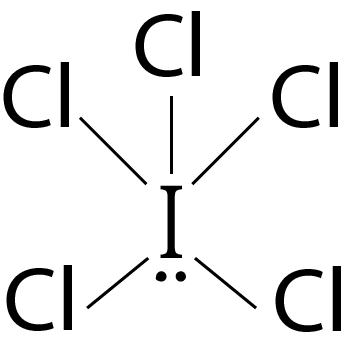ICl4: 4 bond pairs + 2 lone pairs ⟶ sp3d2 hybridisation ⇒  Geometry: Octahedral; Shape :  Square planar shape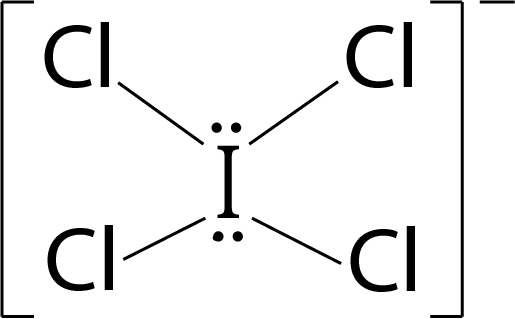### Practice Questions

Question 1:  The bond order and magnetic behaviour of O2 ion are respectively :

1. 1.5 and paramagnetic

2. 1.5 and diamagnetic

3. 2 and diamagnetic

4. 1 and paramagnetic

Question 2: The ion that has sp3d2 hybridisation of the central atom:

are:

1. [ICl2]

2. [ICl4]

3. [IF6]

4. [BrF2]

### How can you prepare for the Chapter of Chemical Bonding?

Even though this chapter is part of physical Chemistry, it is entirely theoretical. It is not necessary to practise formulas or numbers to prepare this chapter. To answer the problems in this chapter, you must first have a thorough understanding of the atomic structure, element classification, and periodicity from the previous chapters. To learn about Chemical Bonding and Molecular Structure, you need to first read its theory from the NCERT book and then work through the examples and questions. Apart from that, you can read O.P Tandon or P. Bahadur's book if you want to prepare for advanced level competitive exams like JEE and NEET. Meanwhile, you can also give mock tests to know your preparation level.

## JEE Main Chemistry Chemical Bonding and Molecular Structure Study Materials

Here, you'll find a comprehensive collection of study resources for Chemical Bonding and Molecular Structure designed to help you excel in your JEE Main preparation. These materials cover various topics, providing you with a range of valuable content to support your studies. Simply click on the links below to access the study materials of Chemical Bonding and Molecular Structure and enhance your preparation for this challenging exam.

 JEE Main Chemical Bonding and Molecular Structure Study Materials JEE Main Chemical Bonding and Molecular Structure Notes JEE Main Chemical Bonding and Molecular Structure Important Questions JEE Main Chemical Bonding and Molecular Structure Practice Paper

## JEE Main Chemistry Study and Practice Materials

Explore an array of resources in the JEE Main Chemistry Study and Practice Materials section. Our practice materials offer a wide variety of questions, comprehensive solutions, and a realistic test experience to elevate your preparation for the JEE Main exam. These tools are indispensable for self-assessment, boosting confidence, and refining problem-solving abilities, guaranteeing your readiness for the test. Explore the links below to enrich your Chemistry preparation.

 JEE Main Chemistry Study and Practice Materials JEE Main Chemistry Previous Year Question Papers JEE Main Chemistry Mock Test JEE Main Chemistry Formula JEE Main Sample Paper JEE Main Chemistry Difference Between

Conclusion:

It is concluded from various theories that the valence shell electrons assist in the bond creation either by sharing electrons or by transferring electrons, according to various theories. The ionic bond generated by electron transfer has some covalent character that can be quantified by Fajan's rule, whereas the covalent bond formed by exchanging electrons has some ionic character.

The topics of chemical bonding and molecular structure are relevant for the JEE competitive examination. This chapter of the examination has a wide range of questions, including easy, medium, and difficult ones. For the purposes of the examination, this article includes all of the significant and important issues.

## FAQs on Chemical Bonding and Molecular Structure Chapter - Chemistry JEE Main

1. Is the chemical bonding chapter important for JEE main?

Yes, chemical bonding is an important chapter for JEE main exam as the questions appearing in the examination paper are of easy level in terms of difficulty. The 2-3  questions from this chapter come in the exam papers.

2. What are the important topics in chemical bonding for JEE main?

The important topics in chemical bonding for JEE main are:

• Lewis dot structures

• Fajan’s rule

• Valence Shell Electron Pair Repulsion (VSEPR) theory

• Resonance Structure

• Molecular Orbital Theory (MOT)

• Magnetic Properties of molecules

• Dipole Moment

• Hydrogen bonding

3. Is chemical bonding there in JEE main?

Yes, chemical bonding is an important topic even for JEE main as it is the basis for the important chapters and topics of chemistry.

4. What are the different types of hybridization?

There are various types of Hybridization. These are sp Hybridization, in which, one s and one p orbitals mix, sp2 Hybridization, in which, ones and two p orbitals mix, sp3 Hybridization, in which, one s and three p orbitals mix, dsp2 Hybridization, in which, ones, two p, and one d orbitals mix, sp3d Hybridization, in which, one s, three p and one d orbitals mix, sp3d2 Hybridization, in which, one s, three p and two d orbitals mix, d2sp3 Hybridization, in which, one s, three p and two d orbitals mix.

5. Can You Justify that an Ionic Bond is Tougher than a Covalent Bond?

An Ionic bond is created from the mutual attraction between differently charged ions but a Covalent Bond is the kind of bond that is created from the distribution of electrons between nuclei.

They tend to be tougher than the covalent bonds as a result of the coulombic attraction between ions of reverse charges.

### Repeaters Course for JEE 2022 - 23## Notice board

JEE News
JEE Blogs